• 2021-12-21 11:39:48

现有一个简易迷宫，如图所示，需要小球从指定位置开始，走到箭头所在位置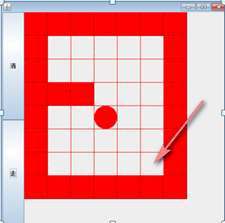接下来是代码实现部分

package com.sanjin.study;

/**
* @author sanjin
* @create 2021-12-20 21:25
*/
public class MiGong {
public static void main(String[] args) {
//创建一个二维数组表示迷宫
int[][] map = new int;
//使用1表示墙
//把上下全部置为1
for (int i = 0; i < 7; i++) {
map[i] = 1;
map[i] = 1;
}
//把左右全部置为1
for (int i = 0; i < 8; i++) {
map[i] = 1;
map[i] = 1;
}
//设置挡板
map = 1;
map = 1;

System.out.println("原始的地图：");
//输出地图
for (int i = 0; i < 8; i++) {
for (int j = 0; j < 7; j++) {
System.out.print(map[i][j] + "\t");
}
System.out.println();
}
//使用递归回溯给小球找路
setWay(map,1,1);
//输出新的地图，小球走过并探测过的
System.out.println("小球走过的路：");
for (int i = 0; i < 8; i++) {
for (int j = 0; j < 7; j++) {
System.out.print(map[i][j] + "\t");
}
System.out.println();
}
}

//使用递归回溯来给小球找路
//说明：
//（i，j）表示小球从哪个位置出发
//如果小球能到达map位置，说明通路找到
//map[i][j]=2，表示通路；map[i][j]=3表示已经走过，但是走不通
//指定策略：下->右->上->左，如果该点走不通，再回溯
public static boolean setWay(int[][] map,int i,int j){
if (map == 2){//通路已经找到
return true;
} else {
if (map[i][j] == 0){//当前点还未走过
//按照下->右->上->左走
map[i][j] = 2;//假定该点可以走通
if (setWay(map,i + 1,j)) {//向下走
return true;
} else if (setWay(map,i,j + 1)){//向右走
return true;
} else if (setWay(map,i - 1,j)){//向上走
return true;
} else if (setWay(map,i,j - 1)){//向左走
return true;
} else {//说明该点是走不通的
map[i][j] = 3;
return false;
}
} else {
return false;
}
}
}
}


造迷宫设挡板没什么可说的，重点在于找路方法采用了递归回溯，小球能否找到指定位置，跟程序员设定的找路方法有很大关系，理解起来不难，但是敲起来真的不好想。

另外有个问题我自己思考了很久，就是小球为什么知道1是挡板，不能走呢？希望能得到各位大佬的解答。

在我把代码打印下来看了很多遍之后，终于捋清了思路。

首先小球被放置在(i,j)点，那么此时的map[i][j]肯定是为0的，所以进入if语句，把此时的(i,j)置换为2，然后开始咱们的下、右、上、左策略。

如果小球的下方，即(i+1,j)位置，是个挡板，那么map(i+1,j)是为1的，进入不了if方法体，直接return false，然后开始判断else if语句，即往右走，如果右边的点，(i,j+1)不是挡板，那么小球走到此点，并把它置为2，就这样一直递归下去，直到找到箭头指示位置。java
更多相关内容
• 首先实现一个以链表作存储结构的栈类型，然后编写一个求解迷宫的非递归程序。求得的通路以 三元组（i，j，d）的形式输出，其中（i，j）指示迷宫中的一个位置（行号和列号），d 表示走到下一 位置的方向（对于迷宫中...
• 数据结构里的迷宫问题，从文件中读取迷宫文件，然后得出解法，存入新的文件c++
• C语言 数据结构中求解迷宫问题实现方法  在学习数据结构栈的这一节遇到了求迷宫这个问题，拿来分享一下~  首先求迷宫问题通常用的是“穷举求解” 即从入口出发，顺某一方向试探，若能走通，则继续往前走，否则...
• #include <stdio.h> #include <malloc.h> #include <stdlib.h> #include <string.h> #include <time.h> #define OK 1 #define ERROR 0 #define NULL 0 #define OVERFLOW -2 #define STACK_INIT_SIZE 100 #define ST
• 已运行过，可自定义迷宫 从键盘输入起点和终点，输出路径。 运用了链栈进行存储，下载即可运行 已运行过，可自定义迷宫 从键盘输入起点和终点，输出路径。 运用了链栈进行存储，下载即可运行 已运行过，可...
• . . . #include <stdio.h> #include <stdlib.h> #include <time.h> #define M 20 #define N 20 #define visited 2 #define TRUE 1 #define FALSE 0 #define INITSIZE 100 typedef int Status; typedef struct{ //...
• //------------ 栈的顺序存储实现 ------------------------------typedef struct...{ int row; int col;}PosType;typedef struct...{ int step; //当前位置在路径上的"序号" PosType seat;...
• 用C++语言实现在迷宫中寻找出路。核心算法伪代码：do{如果当前位置为出口： 当前位置进栈；return 1；while（尝试的方向小于4）{尝试方向号码对应方向的格子；如果这个格子是没走过的通路： 当前位置进栈； 将地图上...
• 本文实例为大家分享了数据结构c语言版迷宫问题栈实现的具体代码，供大家参考，具体内容如下 程序主要参考自严蔚敏老师的数据结构c语言版，在书中程序的大体框架下进行了完善。关于迷宫问题的思路可查阅原书。 #...
• 迷宫问题问题分析 问题分析

# 问题分析

• 用一个二维数组**map表示迷宫的信息，其中‘0’表示可以通过，‘1’表示不可通过，如下图：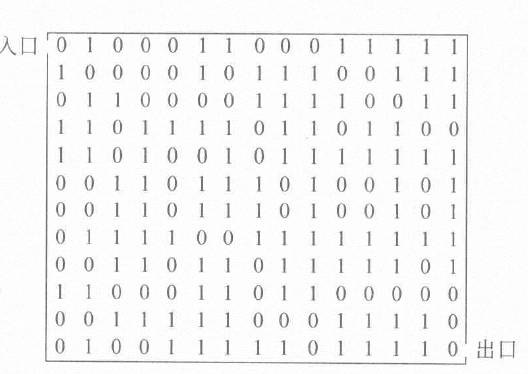• 对于在一个点上的移动方向，可能是东西南北4方向，或者8方向，如下图：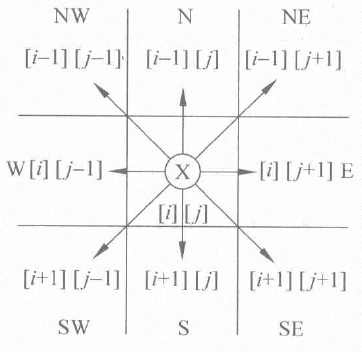• 用一种方法实现找到从出口的到入口的路径。

# 实现方法

## 方向设置

• 我们可以先构建方向结构 o f f s e t s offsets ，用数组 o f f s e t s & m o v e [ 4 ] 或 o f f s e t s & m o v e [ 8 ] offsets\&move或offsets\&move 来表示方向
qmove[q].amove[q].b
N-10
NE-11
E01
SE11
S10
SW1-1
W0-1
NW-1-1
• 代码如下：
//方向枚举:东、西、南、北、东北、东南、西北、西南
enum directions{E,W,S,N,NE,SE,NW,SW};

//表示方向
struct offsets
{
int a, b;
};

//构建移动方向move
//设置方向:选择4方向还是8方向
offsets *move_set(int num = 4)
{
/*方向表示表
___________________________
| q | move[q].a | move[q].b |
-----------------------------
| N |    -1     |     0     |
| NE|    -1     |     1     |
| E |     0     |     1     |
| SE|     1     |     1     |
| S |     1     |     0     |
| SW|     1     |    -1     |
| W |     0     |    -1     |
| NW|    -1     |    -1     |
-----------------------------
*/
offsets* move = new offsets[num];
if (num == 4||num==8)
{

move[N].a = -1;
move[N].b = 0;

move[E].a = 0;
move[E].b = 1;

move[S].a = 1;
move[S].b = 0;

move[W].a = 0;
move[W].b = -1;
}

if (num == 8)
{
move[NE].a = -1;
move[NE].b = 1;

move[SE].a = 1;
move[SE].b = 1;

move[SW].a = 1;
move[SW].b = -1;

move[NW].a = -1;
move[NW].b = -1;
}
return move;
}


## 路径记录和迷宫地图设置

• 建立一个 I t e m s Items 结构，它包括位置坐标 x 和 y x和y 以及下一次该点的移动方向 d i r dir
• 迷宫地图为二维数组，手动输入，代码如下：
//记录路径
struct Items
{
int x, y, dir;
};

//设置地图
char** map_set(const int width, const int high)
{

char** map = new char* [width];
for (int i = 0; i < width; i++)
{
map[i] = new char[high];
}
for (int i = 0; i < width; i++)
{
for (int j = 0; j < high; j++)
{
cin >> map[i][j];
}
}
return map;
}



## 寻找路径

• 首先我们要用数据结构来记录路径信息 ( s t a c k < I t e m s > ∗ w a y s ) (stack<Items>*ways) ，这里之所以用指针是方便后续传参；
• 同时我们构建一个全为零的二维数组 ( c h a r ∗ ∗ m a r k ) (char**mark) 来记录是否已经通过该点，比如通过 x = i , y = j x=i,y=j 的点，那么 m a r k [ i ] [ j ] = 1 mark[i][j]=1
• 话不多说，直接上代码：
//寻找路径
stack<Items>* Path(const int width,const int high,char **map,const char can_pass,const int start_x,const int start_y, const char end_signal,const int move_kind)
{
/*参数说明：
* width:迷宫的长度
* high:迷宫的宽度
* **map:二维迷宫的信息
* can_pass:*map[]中可通过字符
* start_x:起点横坐标
* start_y:起点纵坐标
* end_signal:终点字符标志
* move_kind:4方向移动或8方向移动
*/

//获取方向表示
offsets* move;
move = move_set(move_kind);

//记录走过点的信息
stack<Items>*ways=new stack<Items>;

//记录该点是否走过
int** mark = new int* [width];
for (int i = 0; i < width; i++)
{
mark[i] = new int[high];
for (int j = 0; j < high; j++)
{
mark[i][j] = 0;
}
}

//获取起始点
mark[start_x][start_y] = 1;
Items temp;
temp.x = start_x;
temp.y = start_y;
temp.dir = E;
ways->push(temp);

//开始寻找路径
while (!ways->empty())
{
temp = ways->top();
ways->pop();

int i = temp.x, j = temp.y, d = temp.dir;
while (d < move_kind)
{
int g = i + move[d].a, h = j + move[d].b;
if ((g >= 0 && h >= 0) && (g < width && h < high))
{
if (map[g][h] == end_signal)
{
cout << "exist!" << endl;
// 存储最后一次路径信息
Items temp1;
temp1.x = i;
temp1.y = j;
temp1.dir = E;
ways->push(temp1);
return ways;
}
//可以通过，存储该点
if (map[g][h] == can_pass && mark[g][h]==0)
{
mark[g][h] = 1;
temp.x = i;
temp.y = j;
temp.dir = d + 1;
ways->push(temp);
i = g;
j = h;
d = E;
}
else d++;
}
else d++;
}
}
cout << "not exist!" << endl;
return ways;
}


# 代码总览

#include<iostream>
#include<stack>
using namespace std;

//方向枚举:东、西、南、北、东北、东南、西北、西南
enum directions{E,W,S,N,NE,SE,NW,SW};

//表示方向
struct offsets
{
int a, b;
};

//构建移动方向move
//设置方向:选择4方向还是8方向
offsets *move_set(int num = 4)
{
/*方向表示表
___________________________
| q | move[q].a | move[q].b |
-----------------------------
| N |    -1     |     0     |
| NE|    -1     |     1     |
| E |     0     |     1     |
| SE|     1     |     1     |
| S |     1     |     0     |
| SW|     1     |    -1     |
| W |     0     |    -1     |
| NW|    -1     |    -1     |
-----------------------------
*/
offsets* move = new offsets[num];
if (num == 4||num==8)
{

move[N].a = -1;
move[N].b = 0;

move[E].a = 0;
move[E].b = 1;

move[S].a = 1;
move[S].b = 0;

move[W].a = 0;
move[W].b = -1;
}

if (num == 8)
{
move[NE].a = -1;
move[NE].b = 1;

move[SE].a = 1;
move[SE].b = 1;

move[SW].a = 1;
move[SW].b = -1;

move[NW].a = -1;
move[NW].b = -1;
}
return move;
}

//记录路径
struct Items
{
int x, y, dir;
};

//设置地图
char** map_set(const int width, const int high)
{

char** map = new char* [width];
for (int i = 0; i < width; i++)
{
map[i] = new char[high];
}
for (int i = 0; i < width; i++)
{
for (int j = 0; j < high; j++)
{
cin >> map[i][j];
}
}
return map;
}

//寻找路径
stack<Items>* Path(const int width,const int high,char **map,const char can_pass,const int start_x,const int start_y, const char end_signal,const int move_kind)
{
/*参数说明：
* width:迷宫的长度
* high:迷宫的宽度
* **map:二维迷宫的信息
* can_pass:*map[]中可通过字符
* start_x:起点横坐标
* start_y:起点纵坐标
* end_signal:终点字符标志
* move_kind:4方向移动或8方向移动
*/

//获取方向表示
offsets* move;
move = move_set(move_kind);

//记录走过点的信息
stack<Items>*ways=new stack<Items>;

//记录该点是否走过
int** mark = new int* [width];
for (int i = 0; i < width; i++)
{
mark[i] = new int[high];
for (int j = 0; j < high; j++)
{
mark[i][j] = 0;
}
}

//获取起始点
mark[start_x][start_y] = 1;
Items temp;
temp.x = start_x;
temp.y = start_y;
temp.dir = E;
ways->push(temp);

//开始寻找路径
while (!ways->empty())
{
temp = ways->top();
ways->pop();

int i = temp.x, j = temp.y, d = temp.dir;
while (d < move_kind)
{
int g = i + move[d].a, h = j + move[d].b;
if ((g >= 0 && h >= 0) && (g < width && h < high))
{
if (map[g][h] == end_signal)
{
cout << "exist!" << endl;
// 存储最后一次路径信息
Items temp1;
temp1.x = i;
temp1.y = j;
temp1.dir = E;
ways->push(temp1);
return ways;
}
//可以通过，存储该点
if (map[g][h] == can_pass && mark[g][h]==0)
{
mark[g][h] = 1;
temp.x = i;
temp.y = j;
temp.dir = d + 1;
ways->push(temp);
i = g;
j = h;
d = E;
}
else d++;
}
else d++;
}
}
cout << "not exist!" << endl;
return ways;
}

//打印地图且在地图中显示路径
void Show_path(const int width,const int high,char** map, stack<Items>* ways)
{
if (ways->empty())return;
while (!ways->empty())
{
int x = ways->top().x, y = ways->top().y;
map[x][y] = '@';
ways->pop();
}
for (int i = 0; i < width; i++)
{
for (int j = 0; j < high; j++)
{
cout << map[i][j] << "\t";
}
cout << "\n";
}
}

int main()
{
/*测试数据:(
10 10
#S######.#
......#..#
.#.##.##.#
.#........
##.##.####
....#....#
.#######.#
....#.....
.####.###.
....#...G#
*/
int width, high;
cout << "输入迷宫的长和宽：" << endl;
cin >> width >> high;

char** map;
cout << "输入迷宫信息:" << endl;
map = map_set(width, high);

stack<Items>* ways;
ways = Path(width, high, map, '.', 0, 1, 'G', 4);

Show_path(width, high, map, ways);

return 0;
}


上一节：数据结构-栈与队列–队列

展开全文• ## 数据结构-----迷宫问题（C语言）

千次阅读 多人点赞 2019-10-07 13:36:54
数据结构-----迷宫问题 作者:黑衣侠客 前言 最近学习数据结构中，需要完成老师布置的作业，所以，研究了下迷宫问题，看起来很难的迷宫问题，其实，解决方法有很多，下面我将为大家介绍，用栈是如何解决迷宫问题...

作者:黑衣侠客

# 思路

#### 使用二维数组来对迷宫地图进行编辑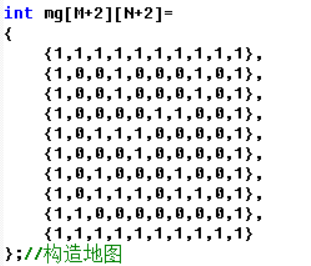#### 之后，我们定义一个栈结构，栈的每一层用来存储走过的每个格子的横坐标，纵坐标，以及从这个格子到下一格子所对应的方向的数字（在这里，我定义的方向是:0-上，1-右，2-下，3-左）,然后再定义一个int型的指针，用于从栈底一次遍历到栈顶，方便对每一层的数据进行操作。具体代码是这样的:

typedef struct
{
int i;              //当前方块的行号
int j;              //当前方块的列号
int di;             //di是下一可走相邻方位的方位号---上下左右的标记
} Box;
typedef struct
{
Box data[MaxSize]; //是用来存储位置坐标的，当MaxSize<位置坐标的数目时，则程序出错，停止运行
int top;            //栈顶指针
} StType;               //定义栈类型

#### 1.定义了mgpath方法，用来执行具体的走迷宫的思想操作

int mgpath(int xi,int yi,int xe,int ye)

#### 2.初始化之前定义的栈，将栈的指针top赋值-1，然后top自增为0，就是栈的第一层，也就是栈底，存放横纵坐标，以及方位值，然后将点所在位置标为-1，以免走重。

 int i,j,k,di,find;
StType st;                  //定义栈st
st.top=-1;                  //初始化栈顶指针
st.top++;                   //初始方块进栈
st.data[st.top].i=xi;//栈底一号存入横坐标数值
st.data[st.top].j=yi;//栈底一号存入纵坐标数值
st.data[st.top].di=-1;//????????????????????????????????
mg[xi][yi]=-1;

#### 3.这是最重要的一步，当栈不是空的时候就一直循环，直到找到终点为止，每次循环开始时，find都为0，而di为0~3，因此，满足条件时，进入内层循环，在内层循环的第一行有一个di++，原因是:每次循环开始时，di的值都为-1，di自增后，就满足了对横纵坐标操作的条件了，我按照上右下左的顺序，改变此时所在的位置坐标，（详解:当di=0时，若走不通，那么就再次进入循环，进行自增，依次类推…），然后，当找到下一个位置为0时（通路），find=1，然后top向上走，在创建一行空间，让每个走过的0位置，都变为-1，此外，需要注意是弹栈操作，当碰到死胡同的时候，那么就需要进行弹栈了，返回到上一步，并将该位置的值从-1变回到0（在进行图像操作时需要用到这一点），（这里不用担心会再次重复走到这个死胡同的位置，因为di++，直接在上一基础方位自增，而不是重新开始，所以不必担心这一点），至此，迷宫思想已全部完成，接下来就该做一些优化了。

while (st.top>-1)           //栈不空时循环
{
i=st.data[st.top].i;
j=st.data[st.top].j;
di=st.data[st.top].di;  //取栈顶方块
if (i==xe && j==ye)     //找到了出口,输出路径
{
printf("迷宫路径如下:\n");
for (k=0; k<=st.top; k++)
{
printf("\t(%d,%d)",st.data[k].i,st.data[k].j);
if ((k+1)%5==0) //每输出5个元素，就换行
printf("\n");
}
printf("\n");
return 0;      //找到路径之后，将所有路径打印出来，然后，程序结束
}
find=0;
while (di<4 && find==0)     //找下一个可走方块
{
di++;
switch(di)
{
case 0://向上走
i=st.data[st.top].i-1;
j=st.data[st.top].j;
break;
case 1://向右走
i=st.data[st.top].i;
j=st.data[st.top].j+1;
break;
case 2://向下走
i=st.data[st.top].i+1;
j=st.data[st.top].j;
break;
case 3://向左走
i=st.data[st.top].i;
j=st.data[st.top].j-1;
break;
}
if (mg[i][j]==0) find=1;    //mg[i][j]==0说明:该点可以走--------------(该点为此时所在点的下一预判点)
}
if (find==1)                    //找到了下一个可走方块
{
st.data[st.top].di=di;      //修改原栈顶元素的di值
st.top++;                   //下一个可走方块进栈
st.data[st.top].i=i;
st.data[st.top].j=j;
st.data[st.top].di=-1;  //再次初始化方向数值
mg[i][j]=-1;                //避免重复走到该方块-----------------------//让每一个点都变成起点，因此，既满足递归的条件，也避免重复走到该位置
}
else                            //没有路径可走,则退栈-----------？？？？？？？？？？？为什么要退栈？不是刚刚只判断了一个方向吗？
{
mg[st.data[st.top].i][st.data[st.top].j]=0;//让该位置变为其他路径可走方块
st.top--;                   //将该方块退栈
}

#### 4.我在主函数中，添加了一些优化操作，主要对输入原点以及图像做了简单处理。

 while(1){
printf("请输入起点坐标:\n");
printf("(横坐标范围:0~9,纵坐标范围:0~9)\n");
scanf("%d %d",&x,&y);
if(mg[x][y]==1){
printf("输入坐标有误，该位置为墙,请重新输入:\n");
}else{
break;
}
}

#### 图像处理：

 printf("\n\n图像表示:\n");
for(t=0;t<10;t++){
printf("\t\t");
for(k=0;k<10;k++){
if(mg[t][k]==1){
printf("#");
}else if(mg[t][k]==0){
printf(" ");
}else{
printf("o");
}
}
if(k==10){
printf("\n");
}
}
printf("\n此时，o所代表的图标为迷宫行走路径!!!\n");

# 代码部分

#include <stdio.h>
#define MaxSize 100
#define M 8
#define N 8
int mg[M+2][N+2]=
{
{1,1,1,1,1,1,1,1,1,1},
{1,0,0,1,0,0,0,1,0,1},
{1,0,0,1,0,0,0,1,0,1},
{1,0,0,0,0,1,1,0,0,1},
{1,0,1,1,1,0,0,0,0,1},
{1,0,0,0,1,0,0,0,0,1},
{1,0,1,0,0,0,1,0,0,1},
{1,0,1,1,1,0,1,1,0,1},
{1,1,0,0,0,0,0,0,0,1},
{1,1,1,1,1,1,1,1,1,1}
};//构造地图
typedef struct
{
int i;              //当前方块的行号
int j;              //当前方块的列号
int di;             //di是下一可走相邻方位的方位号---上下左右的标记
} Box;
typedef struct
{
Box data[MaxSize]; //是用来存储位置坐标的，当MaxSize<位置坐标的数目时，则程序出错，停止运行
int top;            //栈顶指针
} StType;               //定义栈类型
//该栈中:每一个位置用于存储Box，该栈对应了一个int型的top作为指针，依次移动，方便对栈中每一个位置进行操作，而Box中，存储了该迷宫位置的横坐标，纵坐标，和从上一位置移动到该位置所对应的方向数值
int mgpath(int xi,int yi,int xe,int ye) //求解路径为:(xi,yi)->(xe,ye)
//初位置横坐标，初位置纵坐标，终点横坐标，终点纵坐标
{
int i,j,k,di,find;
StType st;                  //定义栈st
st.top=-1;                  //初始化栈顶指针
st.top++;                   //初始方块进栈
st.data[st.top].i=xi;//栈底一号存入横坐标数值
st.data[st.top].j=yi;//栈底一号存入纵坐标数值
st.data[st.top].di=-1;//????????????????????????????????
mg[xi][yi]=-1;
while (st.top>-1)           //栈不空时循环
{
i=st.data[st.top].i;
j=st.data[st.top].j;
di=st.data[st.top].di;  //取栈顶方块
if (i==xe && j==ye)     //找到了出口,输出路径
{
printf("迷宫路径如下:\n");
for (k=0; k<=st.top; k++)
{
printf("\t(%d,%d)",st.data[k].i,st.data[k].j);
if ((k+1)%5==0) //每输出5个元素，就换行
printf("\n");
}
printf("\n");
return 0;      //找到路径之后，将所有路径打印出来，然后，程序结束
}
find=0;
while (di<4 && find==0)     //找下一个可走方块
{
di++;
switch(di)
{
case 0://向上走
i=st.data[st.top].i-1;
j=st.data[st.top].j;
break;
case 1://向右走
i=st.data[st.top].i;
j=st.data[st.top].j+1;
break;
case 2://向下走
i=st.data[st.top].i+1;
j=st.data[st.top].j;
break;
case 3://向左走
i=st.data[st.top].i;
j=st.data[st.top].j-1;
break;
}
if (mg[i][j]==0) find=1;    //mg[i][j]==0说明:该点可以走--------------(该点为此时所在点的下一预判点)
}
if (find==1)                    //找到了下一个可走方块
{
st.data[st.top].di=di;      //修改原栈顶元素的di值
st.top++;                   //下一个可走方块进栈
st.data[st.top].i=i;
st.data[st.top].j=j;
st.data[st.top].di=-1;  //再次初始化方向数值
mg[i][j]=-1;                //避免重复走到该方块-----------------------//让每一个点都变成起点，因此，既满足递归的条件，也避免重复走到该位置
}
else                            //没有路径可走,则退栈-----------？？？？？？？？？？？为什么要退栈？不是刚刚只判断了一个方向吗？
{
mg[st.data[st.top].i][st.data[st.top].j]=0;//让该位置变为其他路径可走方块
st.top--;                   //将该方块退栈
}
}
return(0);                          //表示没有可走路径,返回0
}
int main()
{
int x,y,k,t;
printf("终点坐标为:(8,8)\n");
while(1){
printf("请输入起点坐标:\n");
printf("(横坐标范围:0~9,纵坐标范围:0~9)\n");
scanf("%d %d",&x,&y);
if(mg[x][y]==1){
printf("输入坐标有误，该位置为墙,请重新输入:\n");
}else{
break;
}
}
mgpath(x,y,M,N);
printf("\n\n图像表示:\n");
for(t=0;t<10;t++){
printf("\t\t");
for(k=0;k<10;k++){
if(mg[t][k]==1){
printf("#");
}else if(mg[t][k]==0){
printf(" ");
}else{
printf("o");
}
}
if(k==10){
printf("\n");
}
}
printf("\n此时，o所代表的图标为迷宫行走路径!!!\n");
return 0;
}


# 运行结果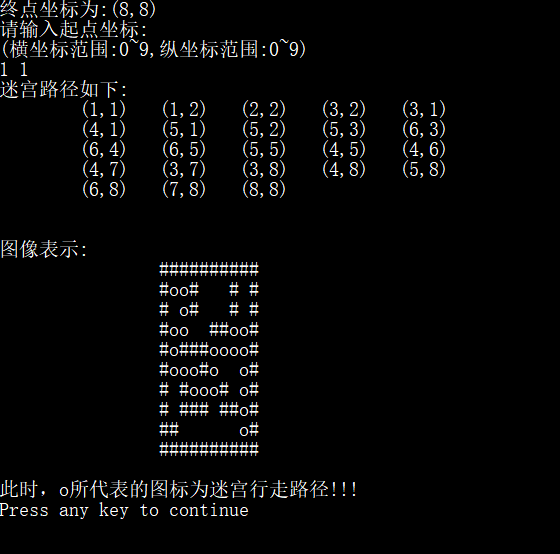#### 本次代码，我已传到github上------迷宫问题

展开全文C语言
• 要求：以书中3.2.4节迷宫求解为基础实现迷宫游戏，游戏运行时显示一个迷宫地图（迷宫内容结构可以参照书中图片，也可以自己编写），玩家从地图左上角的入口处进入迷宫，从右下角出口离开迷宫。玩家不能穿墙而过。本...

一、程序设计思路

1、题目：应用栈实现迷宫游戏

要求：以书中3.2.4节迷宫求解为基础实现迷宫游戏，游戏运行时显示一个迷宫地图（迷宫内容结构可以参照书中图片，也可以自己编写），玩家从地图左上角的入口处进入迷宫，从右下角出口离开迷宫。玩家不能穿墙而过。

2、解决思路

采用“穷举求解”方法,需要用到栈，从入口开始，往四个方向走，依次将走过的坐标点–入栈；如果走到“死路”，就出栈；一一循环；最后栈中保存的就是出迷宫的路线。

3、算法描述

求迷宫中一条从入口到出口的路径的算法可简单描述如下:

设当前位置的初值为入口位置；

do{
若当前位置可通，
则{
将当前位置压至栈顶； //纳入路径
如果当前位置是出口位置，则结束；
否则切换当前位置的东邻方块为新的当前位置；
}
否则，
若栈不为空且栈顶位置尚有其他方向未经探索，
则设定新的当前位置为沿顺时针方向旋转找到的栈顶位置的下一相邻方块；
若栈不为空且栈顶位置的四周均不可通，
则{
删去栈顶位置；
若栈不为空，则重新测试新的栈顶位置，
直至找到一个可通的相邻块或者出栈至栈空；
}
}while(栈不为空)；

二、程序源代码
栈部分



#include "stdafx.h"                //包含标准C的头文件，stdio.h,string.h等

#include <malloc.h>               //动态储存分配函数头文件，用于栈的储存空间分配

#include <stdlib.h>               //standard library标准库头文件

typedef int DirectiveType;        //下一个通道方向

#define RANGE 100                 //迷宫大小

#define STACK_INIT_SIZE 100       //定义栈的初始大小

#define STACKINCREMENT    10      //定义栈的储存增量，在栈长度越界时

typedef int DirectiveType;        //下一个通道方向

#define RANGE 100                 //迷宫大小

#define ROW 10                    //迷宫的行数

#define COL 10                    //迷宫的列数

typedef struct

{

int m, n;

int arr[RANGE][RANGE];       //迷宫数组

}MazeType;                       //迷宫的类型

typedef struct

{

int row;                     //迷宫中的行

int col;                     //迷宫中的列

}PosType;                        //坐标(row,col)

typedef struct

{

int step;                    //当前位置在路径上的"序号"

PosType seat;                //当前的坐标位置

DirectiveType di;            //往下一个坐标位置的方向

}SElemType;                      //栈的元素类型

typedef struct

{

SElemType *base;             //栈底

SElemType *top;              //栈顶

int stacksize;               //栈的大小

}SqStack;                        //定义栈

bool InitStack(SqStack &s)

{                                //栈的初始化

s.base = (SElemType
*)malloc(STACK_INIT_SIZE * sizeof(SElemType));

if (!s.base)

{

exit(-2);

}

s.top = s.base;

s.stacksize = STACK_INIT_SIZE;

return true;

}

bool GetTop(SqStack s, SElemType &e)
//当栈s不为空时,返回栈顶e

{

if (s.top == s.base)

return false;

e = *(s.top - 1);

return true;

}

bool Push(SqStack &s, SElemType e)
//在栈s中插入元素e，入栈

{

if (s.top - s.base >=
s.stacksize)

{                                 //若栈满，追加存储空间

s.base = (SElemType
*)realloc(s.base, (s.stacksize + STACKINCREMENT) * sizeof(SElemType));

if (!s.base)

exit(-2);

s.top = s.base + s.stacksize;

s.stacksize += STACKINCREMENT;

}

*s.top++ = e;

return true;

}

bool Pop(SqStack &s, SElemType &e)     //在栈s中删除栈顶，出栈

{

if (s.top == s.base)               //当栈空时

return false;                  //返回错误

e = *--s.top;                      //e指向新栈顶

return true;

}

bool StackEmpty(SqStack s)
//栈判空

{

return s.base == s.top;

}

bool DestoryStack(SqStack &s)
//销毁栈

{

free(&s);                          //释放栈s的储存空间

return true;

}



迷宫部分



bool InitMaze(MazeType &maze, int a[ROW][COL], int row, int col)  //初始化迷宫

{

int i, j;                            //设置迷宫maze的初值,包括加上边缘一圈的值

for (i = 1; i<row - 1;
i++)

{

for (j = 1; j<col - 1; j++)

{

maze.arr[i][j] = a[i][j];

}

}

for (j = 0; j<row; j++)                     //加上围墙

maze.arr[j] = maze.arr[row
- 1][j] = 1;

for (i = 0; i<col; i++)

maze.arr[i] =
maze.arr[i][col - 1] = 1;

return true;

}

bool Pass(MazeType maze, PosType curpos)

{
//判断当前节点是否通过

if (maze.arr[curpos.row][curpos.col]
== 0)     //当节点为0时返回真

return true;

else

return false;

}

bool FootPrint(MazeType &maze, PosType curpos)

{
//留下足迹

maze.arr[curpos.row][curpos.col]
= 2;           //走过且走得通

return true;

}

bool MarkPrint(MazeType &maze, PosType curpos)

{
//留下不能通过的标记

maze.arr[curpos.row][curpos.col]
= 3;           //走过但走不通

return true;

}

SElemType CreateSElem(int step, PosType pos, int di)

{
//创建元素e

SElemType e;

e.step = step;

e.seat = pos;

e.di = di;

return e;

}

PosType NextPos(PosType curpos, DirectiveType di)   //curpos当前位置

{
//返回当前节点的下一节点

PosType pos = curpos;

switch (di)

{

case 1:        //右

pos.col++;

break;

case 2:        //下

pos.row++;

break;

case 3:        //左

pos.col--;

break;

case 4:        //上

pos.row--;

break;

}

return pos;

}

bool PosEqual(PosType pos1, PosType pos2)

{
//判断是不是出口

if (pos1.row == pos2.row
&& pos1.col == pos2.col)

return true;

else

return false;

}

void PrintMaze(MazeType maze, int row, int col)

{
//打印路径

int i, j;

printf(" ");

for (i = 0; i<col; i++)                    //打印列数名

printf("%d ", i);

printf("\n");

for (i = 0; i<row; i++)

{

printf("%d",
i);                      //打印行数名

for (j = 0; j<col; j++)

{

switch (maze.arr[i][j])

{

case 0:

printf("  ");                 //没走过，但是通路

break;

case 1:

printf("■");                  //墙，障碍物

break;

case 2:

printf("*
");                 //走过且走得通

break;

case 3:

printf("@
");                 //走过但走不通，死胡同

break;

default:

break;

}

}

printf("\n");

}

}

bool MazePath(MazeType &maze, PosType start, PosType end)

{                               //求解迷宫maze中,从入口start到出口end的一条路径

SqStack s;                  //定义栈

SElemType e;

InitStack(s);               //初始化栈

PosType curpos = start;

int curstep = 1;                                //探索第一步

do {

if (Pass(maze, curpos))

{    //如果当前位置可以通过,即是未曾走到的通道块

FootPrint(maze,
curpos);                //留下足迹

e = CreateSElem(curstep,
curpos, 1);    //创建元素

Push(s, e);                             //加入路径

if (PosEqual(curpos,
end))              //到达终点（出口）

return true;

curpos = NextPos(curpos,
1);            //获得下一节点

curstep++;                              //探索下一步

}

else

{                                           //当前位置不能通过

if (!StackEmpty(s))

{

Pop(s, e);

while (e.di == 4
&& !StackEmpty(s)) //找寻了四个方向

{

MarkPrint(maze,
e.seat);

Pop(s, e);                      //留下不能通过的标记,并退回一步

}

if (e.di<4)

{

e.di++;                         //换一个方向探索

Push(s, e);

curpos =
NextPos(e.seat, e.di); //设定当前位置是该方向上的相邻块

}

}

}

} while (!StackEmpty(s));

return false;

}



主函数部分



int main()                   //迷宫求解主函数

{

int i, j;

PosType start, end;      //开始，终点坐标

MazeType maze;

int a[ROW][COL] = {                 //原始迷宫，其中'1'表示墙，'0'表示通道

{ 1,1,1,1,1,1,1,1,1,1 },

{ 1,0,0,1,0,0,0,1,0,1 },

{ 1,0,0,1,0,0,0,1,0,1 },

{ 1,0,0,0,0,1,1,0,0,1 },

{ 1,0,1,1,1,0,0,0,0,1 },

{ 1,0,0,0,1,0,0,0,0,1 },

{ 1,0,1,0,0,0,1,0,0,1 },

{ 1,0,1,1,1,0,1,1,0,1 },

{ 1,1,0,0,0,0,0,0,0,1 },

{ 1,1,1,1,1,1,1,1,1,1 }

};

printf("\n-------------------------------------------------\n");

printf("\n原始迷宫如下：\n");

printf("(其中'1'表示墙，'0'表示通道)\n");

for (i = 0; i<10; i++)           //双重循环输出原始迷宫

{

for (j = 0; j<10; j++)

{

printf("%d ",
a[i][j]);

}

printf("\n");

}

InitMaze(maze, a, ROW, COL);                 //初始化迷宫

start.row = 1;                               //给定迷宫起点坐标

start.col = 1;                               //（1，1）

end.row = 8;                                 //给定迷宫终点坐标

end.col = 8;                                 //（8，8）

if (MazePath(maze, start,
end))              //如果找到一条路径

{

printf("\n-------------------------------------------------\n");

printf("\n穷举法求解迷宫路径如下：\n");

printf("(其中'*'表示求解路径，'@'表示死胡同)\n");

PrintMaze(maze, ROW,
COL);               //输出迷宫路径

}

else                                         //否则，没有通路

printf("\n---------从入口到出口没有通路!-----\n");

}



三、运行结果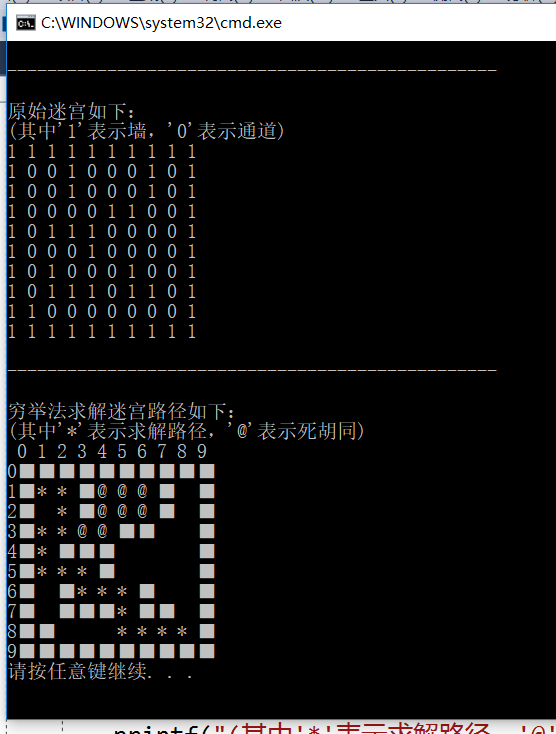附：源代码链接迷宫求解源代码

展开全文C语言
•C++ 算法
• 迷宫问题——数据结构课程设计迷宫问题完整版(含源代码).docx迷宫问题——数据结构课程设计迷宫问题完整版(含源代码).docx迷宫问题——数据结构课程设计迷宫问题完整版(含源代码).docx迷宫问题——数据结构课程设计...
• 迷宫问题——数据结构课程设计迷宫问题完整版(含源代码).pdf迷宫问题——数据结构课程设计迷宫问题完整版(含源代码).pdf迷宫问题——数据结构课程设计迷宫问题完整版(含源代码).pdf迷宫问题——数据结构课程设计迷宫...
• 用栈来求解迷宫数据结构实验。求解迷宫代码来自书上，迷宫只能在源代码上修改。
•python 开发语言 后端
• 代码文件为txt文件，其它所有的均为.c文件，不同于一些只提供无用链接不同。
• 这里写自定## 标题义目录标题欢迎使用Markdown编辑器新的改变功能快捷键合理的创建标题，有助于目录的生成如何改变文本的样式插入链接与图片如何插入一段漂亮的代码片生成一个适合你的列表创建一个表格设定内容居中...c++
• 设计题目: 迷宫问题求解 问题描述 迷宫问题是取自心理学的一个古典实验在该实验中把一只老鼠从一个无顶大盒子的门放入在盒子中设置了许多墙对行进方向形成了多处阻挡盒子仅有一个出口在出口处放置一块奶酪吸引老鼠...
• 迷宫问题,代码如下： package main import ( "fmt" ) //编写一个函数，完成老鼠找路 //myMap *int:地图，保证是同一个地图，使用引用 //i,j 表示对地图的哪个点进行测试 func SetWay(myMap *int, i ...Go语言
• 迷宫实验是取自心理学的一个古典实验。在该实验中，把一只老鼠从一个无顶大盒子的门放入，在盒中设置了许多墙，对行进方向形成了多处阻挡。盒子仅有一个出口处放置一块奶酪，吸引老鼠在迷宫中寻找道路以到达出口。对...
• 程序开始运行时显示一个迷宫地图，迷宫中央有一只老鼠，迷宫的右下方有一个粮仓。游戏的任务是使用键盘上的方向键操纵老鼠在规定的时间内走到粮仓处。 设计要求： （1）老鼠形象可辨认，可用键盘操纵老鼠上下左右...
• 数据结构中的C语言版迷宫代码。 已调试运行过。 绝对真实。
• 迷宫问题是典型的栈的应用实例，具体算法伪代码如下 我是自己定义了栈的一个类，如果觉得麻烦，C++为程序员提供了库文件，输入#include就可以调用以下函数 完整代码 #include <iostream> using namespace st
• 本文针对数据结构基础系列网络课程(3)：栈和队列中第6课时栈的应用2-迷宫问题。例：求出从入口到出口的路径 程序实现：#include #define MaxSize 100 #define M 8 #define N 8 int mg[M+2][N+2]= { {1,1,1,1,1,1,...结构...

# 数据结构迷宫问题代码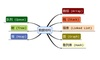数据结构 订阅